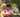### Number Of Disc Intersections

January 28, 2020

Number Of Disc Intersections

We draw N discs on a plane. The discs are numbered from 0 to N − 1. An array A of N non-negative integers, specifying the radiuses of the discs, is given. The J-th disc is drawn with its center at (J, 0) and radius A[J].

We say that the J-th disc and K-th disc intersect if J ≠ K and the J-th and K-th discs have at least one common point (assuming that the discs contain their borders).

The figure below shows discs drawn for N = 6 and A as follows:
A = 1
A = 5
A = 2
A = 1
A = 4
A = 0

There are eleven (unordered) pairs of discs that intersect, namely:
• discs 1 and 4 intersect, and both intersect with all the other discs;
• disc 2 also intersects with discs 0 and 3.

Write a function:
function solution(A);
that, given an array A describing N discs as explained above, returns the number of (unordered) pairs of intersecting discs. The function should return −1 if the number of intersecting pairs exceeds 10,000,000.

Given array A shown above, the function should return 11, as explained above.

Write an efficient algorithm for the following assumptions:
• N is an integer within the range [0..100,000];
• each element of array A is an integer within the range [0..2,147,483,647].

Hints:

• convert 2D discs into 1D line segments on X axis into touples with Left-x and Right-x ([x0, x1])
• sort the touples by Left-x (x0)
• add counters if next segment's Left-x <= previous segment's Right-x
``````function solution(A) {
// write your code in JavaScript (Node.js 8.9.4)
//console.log(A)
//[1, 5, 2, 1, 4, 0]

// convert 2D discs into 1D line segments on X axis
let tupples = []
let i
let j

for(i=0; i<A.length; i++){
tupples.push( [ i-A[i], i+A[i] ] )
}
//console.log("source: ",tupples)
//[ [ -1, 1 ], [ -4, 6 ], [ 0, 4 ], [ 2, 4 ], [ 0, 8 ], [ 5, 5 ] ]

tupples.sort((a,b)=>a-b)
//console.log("sorted: ", tupples)
//[ [ -4, 6 ], [ -1, 1 ], [ 0, 4 ], [ 0, 8 ], [ 2, 4 ], [ 5, 5 ] ]

let count = 0
for(i=0; i<A.length; i++){
for(j=i+1; j<A.length; j++){
if(tupples[j] <= tupples[i]){
// next j segment start point is inside i segment
count ++
if(count>10000000) return -1
}else{
break
// next j segments are not inside i segment
}
}
}

return count
}``````Posted by Tai Lu ( 呂台生 ) who's building useful things.You should follow him on Twitter